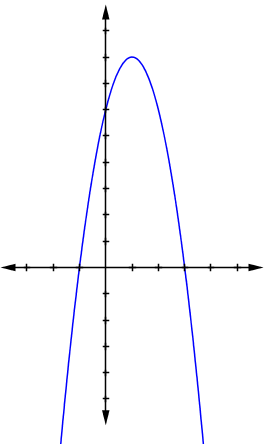### Home > GC > Chapter 12 > Lesson 12.2.2 > Problem12-67

12-67.

On graph paper, make a table and graph the function $f\left(x\right)=-2\left(x-1\right)^2+8$.

Make sure to make a table of points.1. Label the $x$- and $y$-intercepts and state their coordinates.

Where does the graph cross the $x$-axis? The $y$-axis?

2. Name the vertex.

$(1, 8)$

3. Find $f\left(100\right)$ and $f\left(-15\right)$.

Substitute these values into the equation.